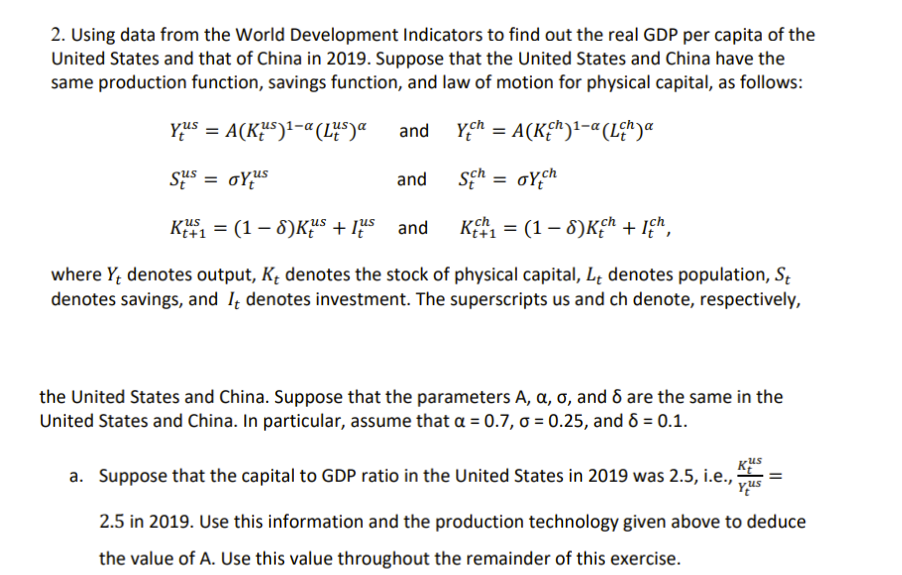### Create an Account

Home / Questions / Using data from the World Development Indicators to find out the real GDP per capita of t...

# Using data from the World Development Indicators to find out the real GDP per capita of the United States and that of China in 2019. Suppose that the United States and China have the same production

Using data from the World Development Indicators to find out the real GDP per capita of the United States and that of China in 2019. Suppose that the United States and China have the same production function, savings function, and law of motion for physical capital, as follows: Yius = A(KUS)1-a (L4S) a and ych = A(K)1-a (Lěh)a Sus = oyus and sch = orch K41 = (1 -8K4s + lus and Kch = (1 -8Kch + ich, where Yt denotes output, Kt denotes the stock of physical capital, Lt denotes population, St denotes savings, and 14 denotes investment. The superscripts us and ch denote, respectively, the United States and China. Suppose that the parameters A, a, o, and 8 are the same in the United States and China. In particular, assume that a = 0.7, o = 0.25, and 8 = 0.1. a. Suppose that the capital to GDP ratio in the United States in 2019 was 2.5, i.e., kus Yus 2.5 in 2019. Use this information and the production technology given above to deduce the value of A. Use this value throughout the remainder of this exercise.Show transcribed image text

Jun 03 2021 View more View LessSubscribe To Get Solution